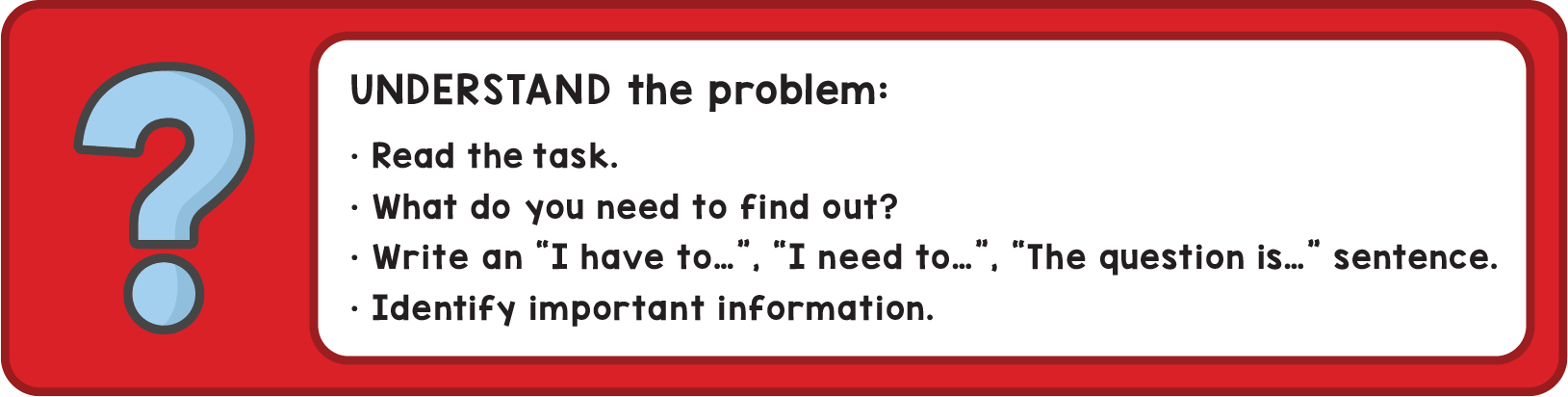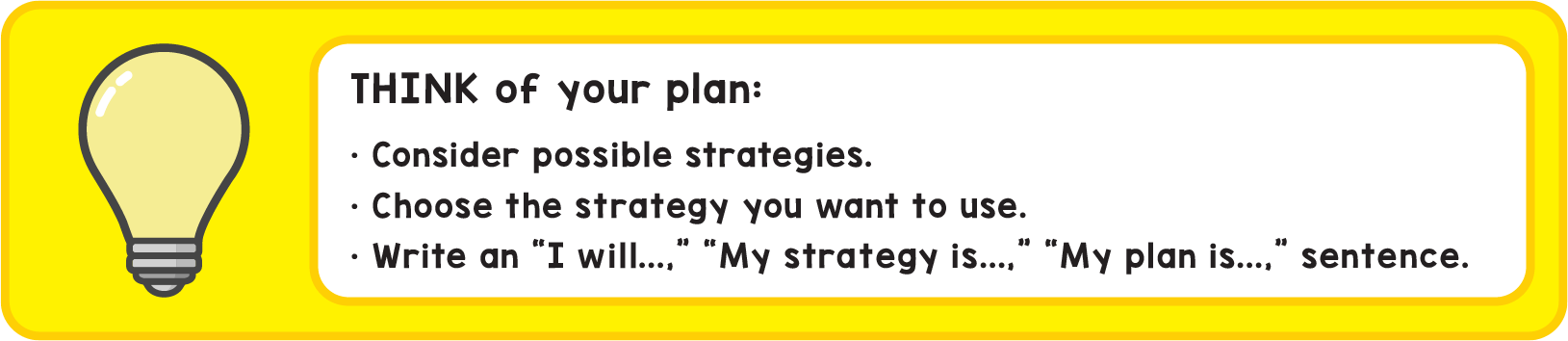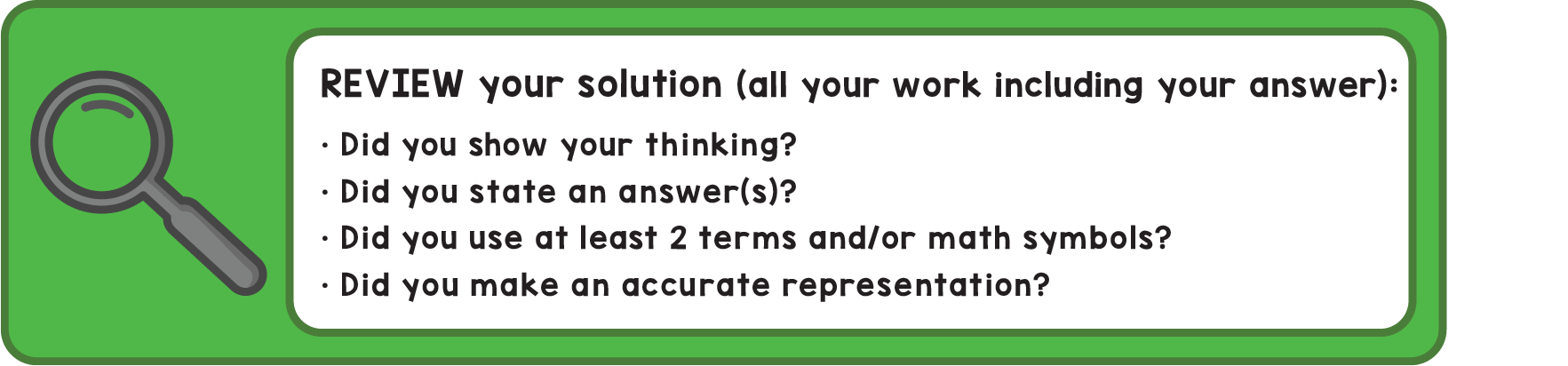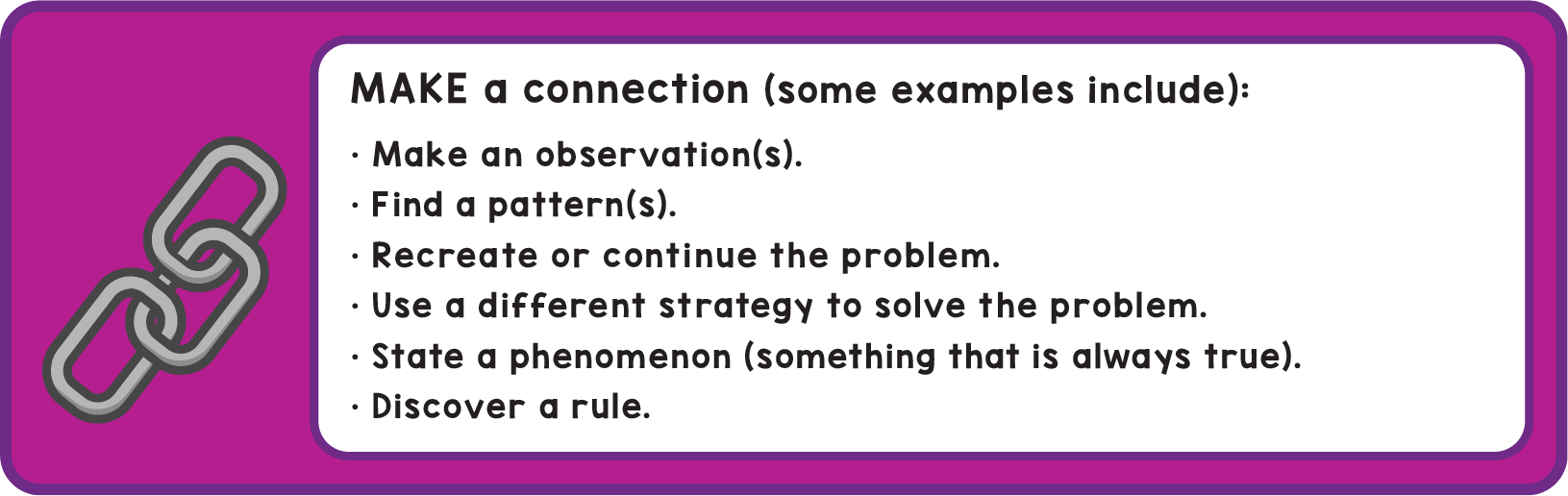# What Does A Strong Solution Look Like?

Upon completing an Exemplars task, what should a student’s final problem-solving solution look like? What should be included? In this video, we will walk students through what they should be thinking about and what they should include to create a strong final solution.

The goal of completing an Exemplars problem-solving task is more than just arriving at a correct answer. Students need to show all of their mathematical thinking so that any reader can understand their strategy. Students also need to prove their thinking and walk any reader through their thinking process. The Exemplars Problem Solving Procedure and Student Rubric guide students through this process.

A Complete Solution

First, students need to provide an “I have to...” sentence, explaining what the problem is asking to be solved, in the student’s own words. This is asked for in the red section of the Problem Solving Procedure.Second, students need to describe the strategy they will use to solve the problem. For this, students should write an “I will ...” sentence, or “My strategy is …” For example: “I will use a diagram” or “I will use a bar graph” (table, graph, etc). This is indicated in the yellow section of the Problem Solving Procedure sheet.Third, students should show the actual math strategy–a diagram, a number line, a table, a graph, etc.--they selected to solve the problem. Whatever their strategy, students need to show this within their work. This is also where students can include their calculations, equations, formulas, etc. A question for students to be thinking about is, “What calculations does a reader need to see to trust that the answer I provided is correct?” This is the blue section of the Problem Solving Procedure sheet.Fourth, students should use the Problem Solving Procedure or Student Rubric to review their solutions and “self-assess” their work.

• Did the student state an answer?
• Did the the student include at least two formal math terms and symbolic notation beyond basic numerals and calculation symbols?
• Are the representations appropriate, labeled and accurate?

This is the green section of the colored Problem Solving Procedure sheet.Finally, students need to include a mathematical connection. Connecting today’s math thinking with ideas students have learned in the past helps build a rich understanding of how all math concepts fit together. Students can make a connection at any time while creating their solution. Some connections could include:

• How does this problem connect with the math they have been studying all year?
• Can the students use another representation to solve the problem?
• Can the student solve the problem another way?
• Does the student see any patterns?

This is the purple/chain section of the colored Problem Solving Procedure sheet.We hope this video will help students develop rich, clear solutions. Enjoy! And, please let us know if you have any questions. Be well!

Topics: